Highest Common Factor

Chapter 3 Class 6 Playing with Numbers
Concept wise

HCF means highest common factor . It means the greatest number which can divide the given numbers

HCF is also known as Greatest Common Divisor (GCD)

#### Find HCF of 4 & 6

To find HCF , we do prime factorization of 4 & 6 separately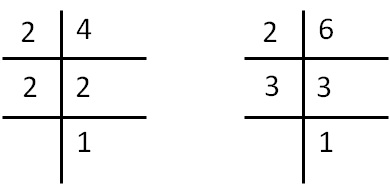4 = 2 × 2

6 = 2 × 3

HCF of 4 & 6 = 2

#### Find HCF of 12 & 16

To find HCF , we do prime factorization of 12 & 16 separately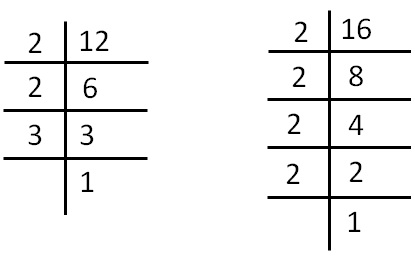12 = 2 × 2 × 3

16 = 2 × 2 × 2 × 2

HCF of 12 & 16 = 2 × 2 = 4

#### Find HCF of 20, 28, 36

Doing Prime factorization of 20, 28 & 36 separately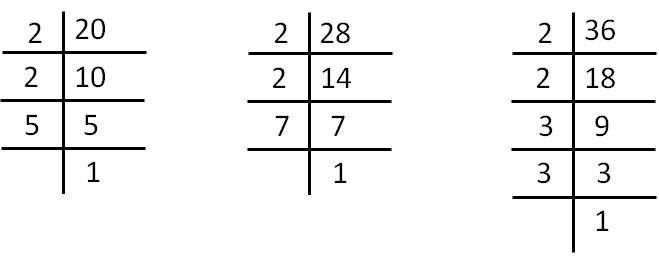20 = 2 × 2 × 5

28 = 2 × 2 × 7

36 = 2 × 2 × 3 × 3

So, HCF of 20, 28 & 36 = 2 × 2 = 4

#### HCF of 13 & 23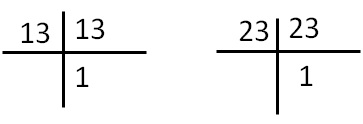13 = 13

23 = 23

Since there are no common prime factors ,

only 1 will be the common factor

∴ HCF = 1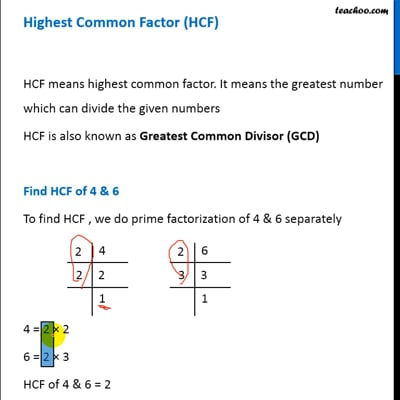This video is only available for Teachoo black users